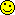# Math Is Fun Forum

Discussion about math, puzzles, games and fun.   Useful symbols: ÷ × ½ √ ∞ ≠ ≤ ≥ ≈ ⇒ ± ∈ Δ θ ∴ ∑ ∫  π  -¹ ² ³ °

You are not logged in.

## #1 2023-04-07 07:31:05

genabutov
Novice
Registered: 2023-03-25
Posts: 4

### Ellipses and hyperbolas of decompositions of even numbers into primes.

1. The sum of two prime numbers is represented as an ellipse equation. A hypothesis is put forward about the infinity of intersecting ellipses given in the form of equations with prime numbers. The conditions for the intersection of ellipses are determined when decomposing even numbers into two primes.
2. The differences of two prime numbers are represented as an equation of a hyperbola. A hypothesis is put forward about the infinity of intersecting hyperbolas, which are given in the form of equations with prime numbers. The conditions for the intersection of hyperbolas are determined when decomposing even numbers into two primes.

Offline

## #2 2023-04-08 01:17:41

BobRegistered: 2010-06-20
Posts: 9,718

### Re: Ellipses and hyperbolas of decompositions of even numbers into primes.

hi genabutov

Would you give one or more examples of what this is about please.

Bob

Children are not defined by school ...........The Fonz
You cannot teach a man anything;  you can only help him find it within himself..........Galileo Galilei
Sometimes I deliberately make mistakes, just to test you!  …………….BobOffline

## #3 2023-04-08 02:50:03

genabutov
Novice
Registered: 2023-03-25
Posts: 4

### Re: Ellipses and hyperbolas of decompositions of even numbers into primes.

hi Bob, here is a link to an article on this topic https://drive.google.com/file/d/1CBQyNyKmOlU9k6oP_Wp1wx2t3TXBbnKi/view

Offline

## #4 2023-04-08 22:14:54

BobRegistered: 2010-06-20
Posts: 9,718

### Re: Ellipses and hyperbolas of decompositions of even numbers into primes.

hi genabutov

Many thanks.  So that's your article?

I've tried 2n = 4, 6, 8, 10.  So far so good.

There are two pairs of primes here, 5 + 13, and 7 + 11

Different ellipses so they intersect.  Is that what you mean?

pmin is 5 and pmax is 13

It seems to me that you'll always get an ellipse if you construct an equation of the form

I haven't worked down to your hypothesis yet.

Whether this is always a 'thing' depends on Goldbach.  Maybe you'd like to prove that on the way.Or perhaps you could turn the whole thing around and use ellipses of this form to prove Goldbach.  Now that would be a thing!!

Need a while to 'digest' the rest.Bob

ps. I've moved the thread out of science HQ and into 'This is Cool' area.

Children are not defined by school ...........The Fonz
You cannot teach a man anything;  you can only help him find it within himself..........Galileo Galilei
Sometimes I deliberately make mistakes, just to test you!  …………….BobOffline

## #5 2023-04-09 07:22:23

genabutov
Novice
Registered: 2023-03-25
Posts: 4

### Re: Ellipses and hyperbolas of decompositions of even numbers into primes.

This is just an attempt to associate sums or differences of prime numbers with points lying on an ellipse or hyperbola.
Certain pairs of prime numbers can be represented as radius-distances from the focuses to points lying either on the ellipse or on the hyperbola.

Equations of the ellipse: |p(k)| + |p(t)| = 2*n

Equations of the hyperbola: ||p(k)| - |p(t)|| = 2*n

where p(k) and p(t) are prime numbers (p(1) = 2, p(2) = 3, p(3) = 5, p(4) = 7,...),
k and t are indices of prime numbers,
2*n is a given even number,
k, t, n ∈ N.

If we construct ellipses and hyperbolas based on the above, we get the following:

1. Hypothesis of intersecting decomposition ellipses: there are only 5 non-intersecting curves (for 2n=4; 2n=6; 2n=8; 2n=10; 2n=16). The remaining ellipses have intersection points.

2. Hypothesis of intersecting decomposition hyperbolas: there is only 1 non-intersecting hyperbola (for 2n=2) and 1 non-intersecting vertical line. The remaining hyperbolas have intersection points.

Offline

## #6 2023-04-12 03:32:23

BobRegistered: 2010-06-20
Posts: 9,718

### Re: Ellipses and hyperbolas of decompositions of even numbers into primes.

Apologies if the following is obvious and I should know it but two thoughts occur to me.

(1) What about non primes? Is what you are saying only apllicable to primes.

(2) I can see it's relatively easy to 'prove' the small number cases and hence deduce they are non intersecting.  But is it always true that higher values are guaranteed to produce interescting cases.  Can you prove this?

Bob

Children are not defined by school ...........The Fonz
You cannot teach a man anything;  you can only help him find it within himself..........Galileo Galilei
Sometimes I deliberately make mistakes, just to test you!  …………….BobOffline

## #7 2023-04-12 04:01:19

genabutov
Novice
Registered: 2023-03-25
Posts: 4

### Re: Ellipses and hyperbolas of decompositions of even numbers into primes.

1. I specifically began to consider ellipses and hyperbolas generated by sets of pairs of prime numbers.
I really wanted neighboring ellipses/hyperbolas to intersect in pairs - it would be nice and simple.
But the prime numbers again presented an unexpected surprise.
I noticed another peculiarity - not all neighboring (closest to each other) ellipses/hyperbols intersect each other.

2. The fact is that I didn't even prove anything. I have only put forward 2 hypotheses.
That is, my whole article is just a statement of the problem, not its solution.
The calculations that I have given are most similar to a computational experiment that shows a limited number of results does not cover the entire infinite set.
Someday someone will prove these two hypotheses.

Offline